# 1.本章学习总结

## 1.1思维导图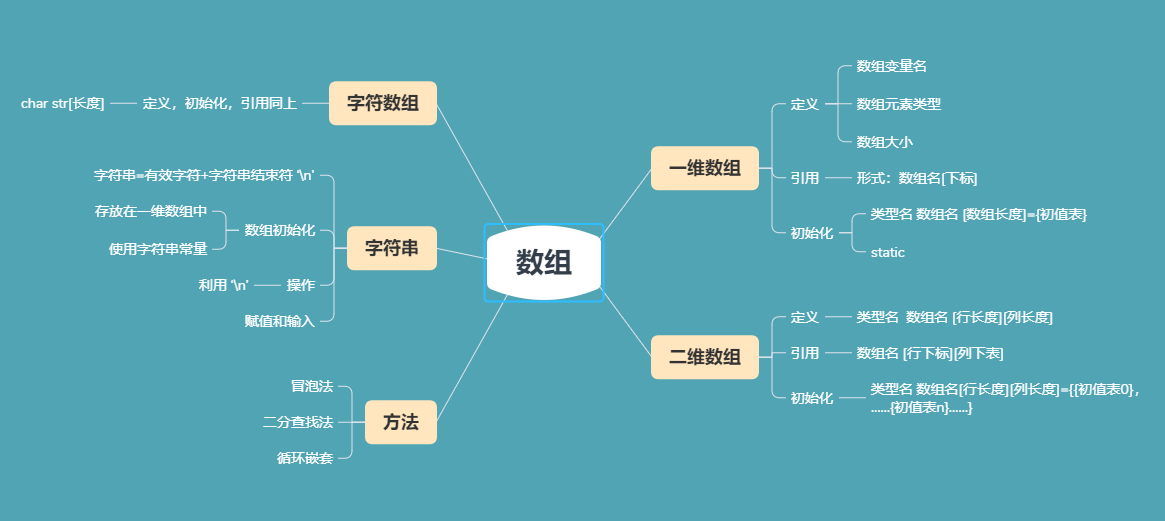## 1.2 本章学习体会及代码量学习体会

### 1.2.2代码累计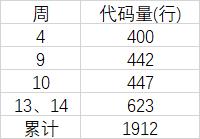# 2.PTA总分

## 2.1截图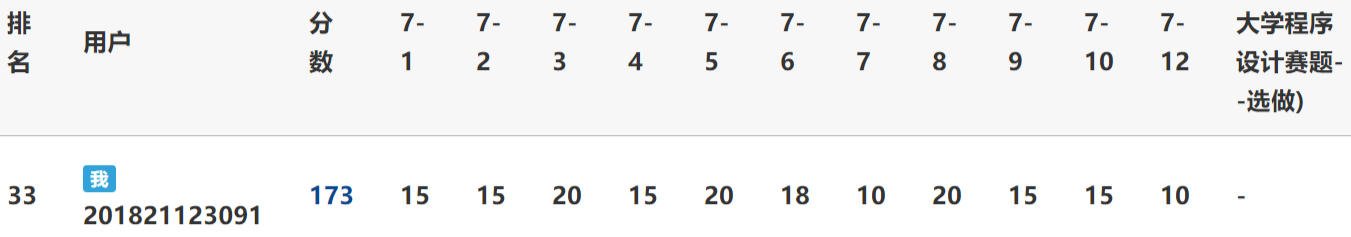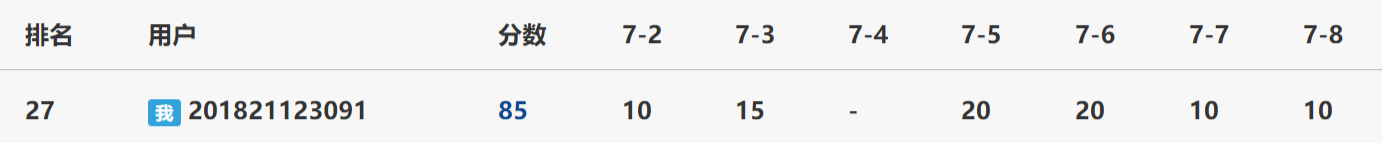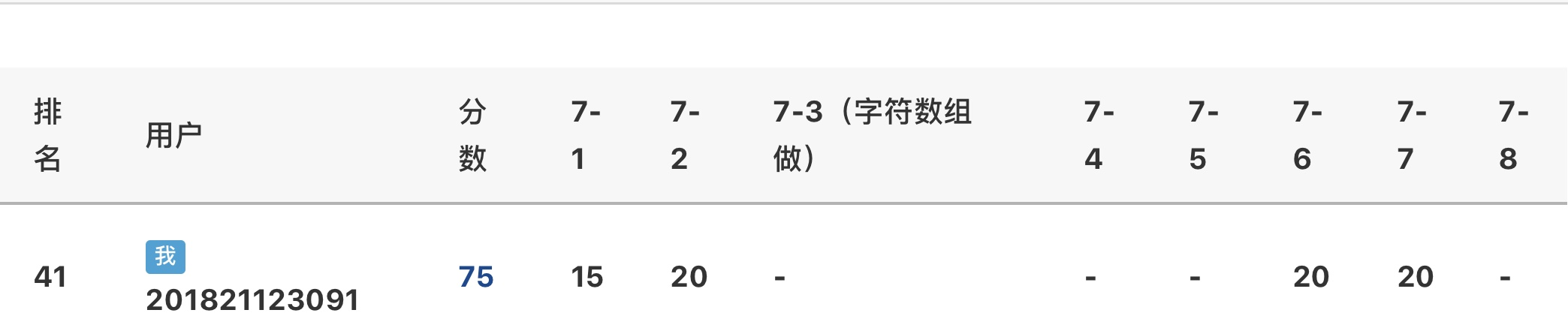335

# 3.PTA实验作业

## 3.1PTA题目一

### 3.1.1算法分析

定义N与整形数组a

for i=0 to N do
flag=m;
m=0;
for j=i to 0 do
if a[i]==a[j] then m++;
end if
if m>flag then
次数为m；
出现次数最多的整数num=a[i];
end if



### 3.1.2代码截图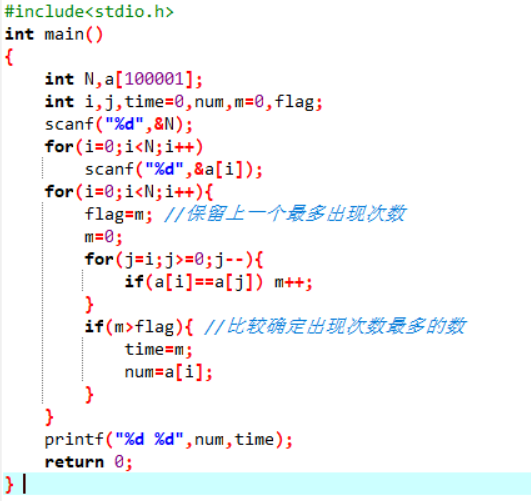### 3.1.3PTA提交列表及说明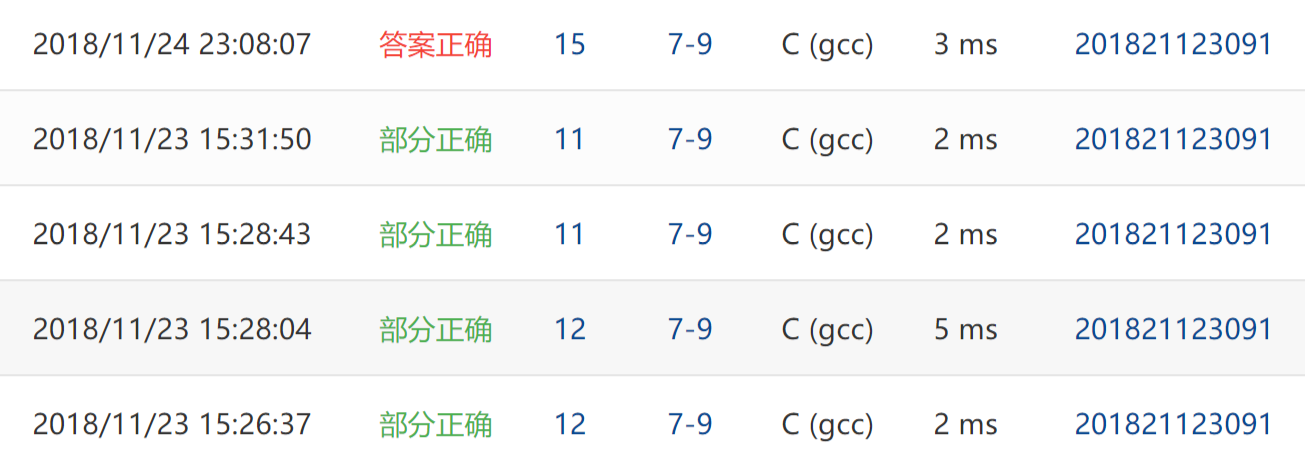• Q1 刚开始是将输入数字作为元素下标，输入该数字时，相应的元素大小加一。最后比较元素大小。但是这种做法在pta中有个测试点一直过不去。怎么改范围都不对。问了老师，说是范围越界，这种方法应该不行。

• A1 最后换了方法：计算每个数出现的次数，然后比较。最后对了。

## 3.2PTA题目二

### 3.2.1算法分析

定义 n,hang,lie

for i=0 to n-1 do
max=a[i]
for j=0 to n-1 do
if a[i][j] 大等于 max then
lie=j;
end if
min=a[lie];
for k=0 to n-1 do
if a[k][lie] 小等于 min then
hang=k;
end if
if min等于max then
输出hang和lie，结束循环
if i>=n then 输出NONE
end



### 3.2.2代码截图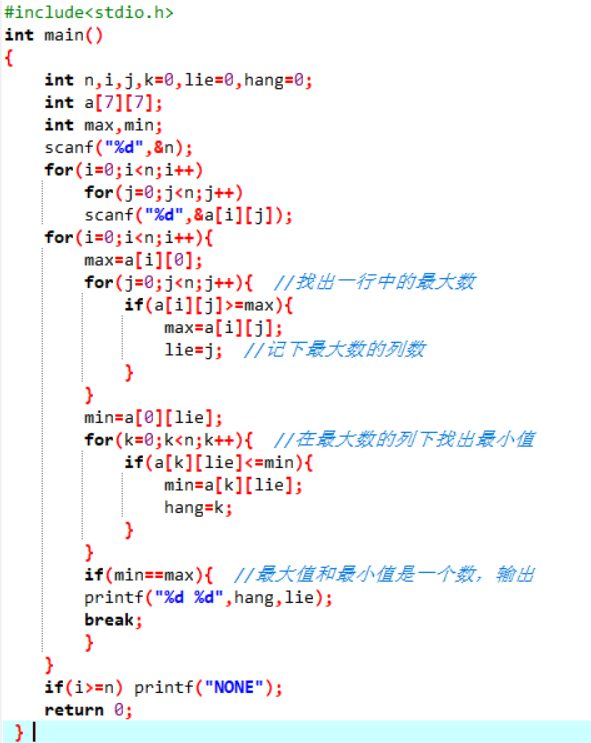### 3.2.3PTA提交列表及说明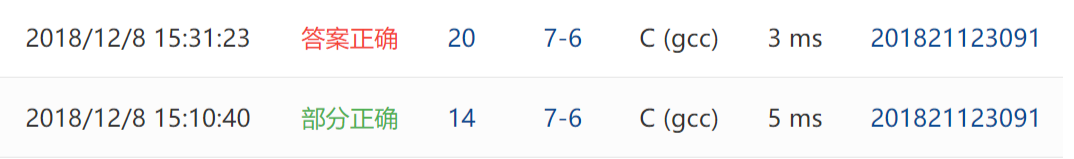• Q1 第一次编写的时候，把行的最大值和列的最小值分开求了。

• A1 改为先求一行中的最大值，再在相应的列中找最小值。

• Q2 循环的嵌套的两个条件写反了，把行和列搞反了。

• A2 理清思路重新改了过来。

• Q3 冒泡法的使用混乱。

• A2 通过上网查询弄清楚了。

# 3.3PTA题目三

### 3.3.1算法分析

定义两个字符数组str,a,定义geshi,flag

gets(str)
geshu=strlen(str);
for i=0 to geshu-1 do
if str[i]是数字字符 || str[i]是大写字母字符 || str[i]是小写字母字符 then
a[j]=str[i];
j++;
end if
if j==0 且 str[i]等于'-' then flag=1;
for i=j-1 to i>=0 do
if a[i]是大写字母字符 then Decnum=Decnum+(a[i]-55)*pow(16,j-1-i);
else if a[i] 是小写字母字符 then Decnum=Decnum+(a[i]-87)*pow(16,j-1-i);
else Decnum=Decnum+(a[i]-'0')*pow(16,j-1-i);
if flag==1 then Decnum=(-1)*Decnum;
printf("%d",Decnum);
end



### 3.3.2代码截图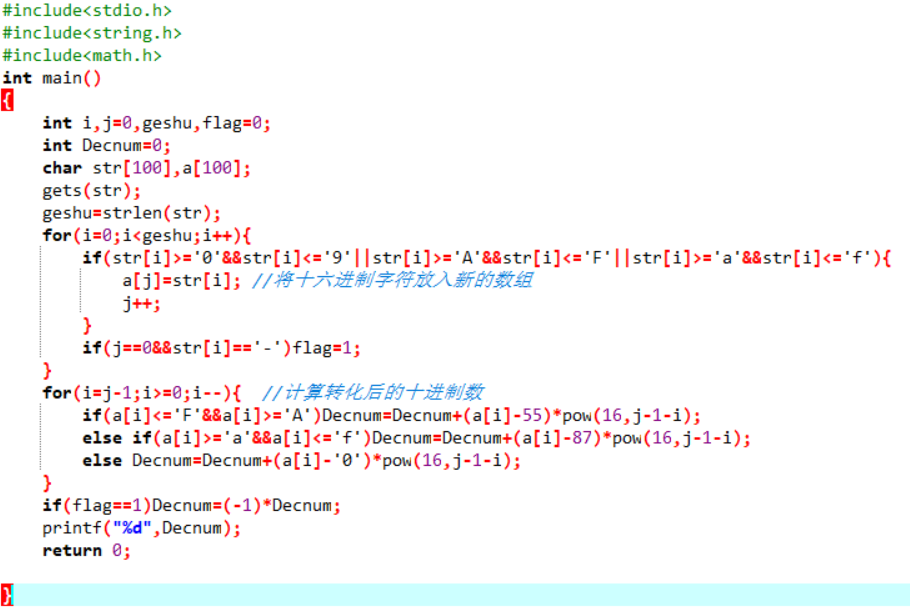### 3.3.3PTA提交列表及说明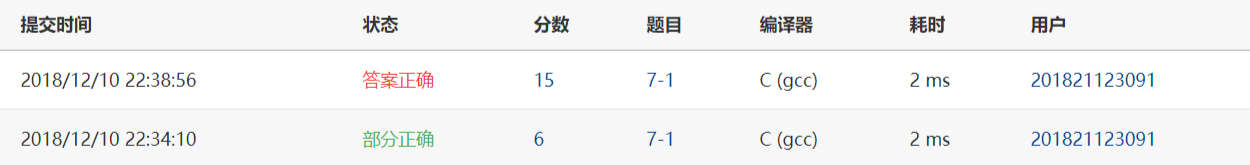• Q1 刚开始想这道题目的时候没有头绪，不知道怎么计算字符个数

• A1 后来经提醒，知道了可以用strlen函数。

• Q2 在小写字符的转化这块卡了很久，一直不知道怎么会转化错误。

• A2 百度后了也找不到错误元因，后来把它前面的if语句改成else if再放到后面就行了。

# 4.代码互评

## 4.1代码截图

• 同学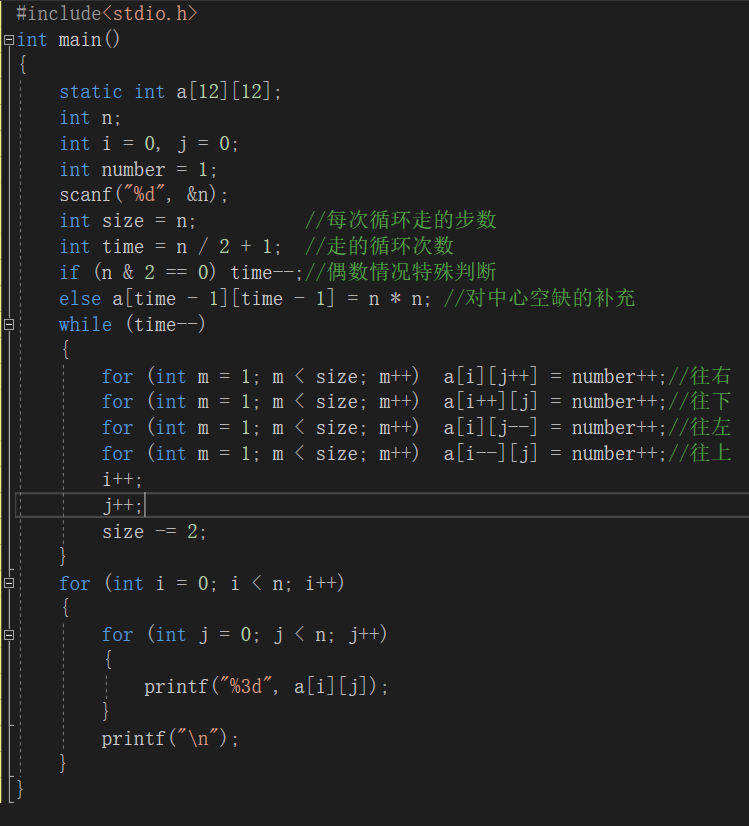• 自己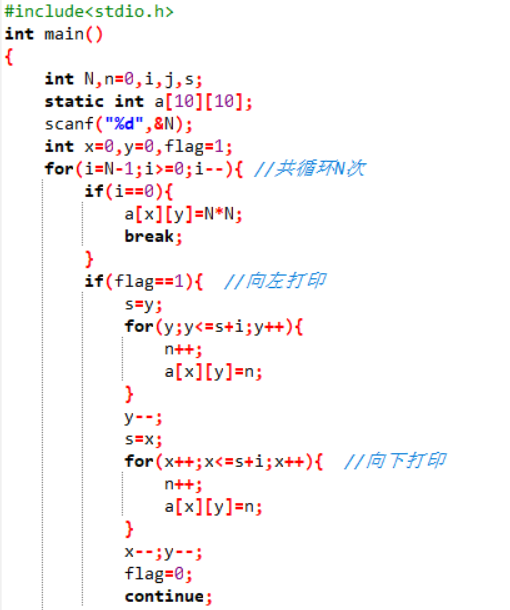## 4.2二者的异同

• 1.同学的思路是设定打印一圈为一个循环，循环次数是n/2+1,因为n为奇数个时，中间正好是镂空，另行补充。我的思路是向左，向下打印和向右，向上打印交错进行，循环次数刚好是N。
• 2.对方的代码比我的简洁很多，条理清晰，可读性强。而自己的代码在编辑的过程中容易出错。
• 3.对方的代码运行速度应该也比我的快。
posted @ 2018-12-08 22:47  chianun  阅读(1208)  评论(0编辑  收藏  举报